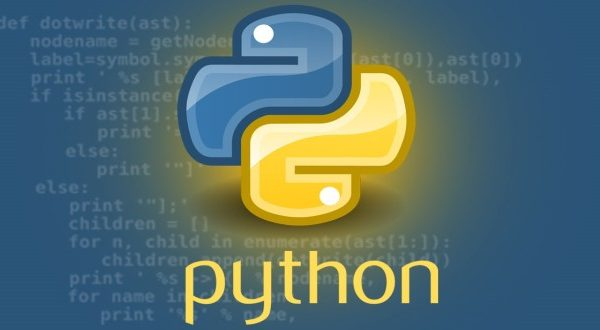# Matplotlib Scatter Plot with Distribution Plots (Joint Plot) – Tutorial and Examples### Introduction

There are many data visualization libraries in Python, yet Matplotlib is the most popular library out of all of them. Matplotlib’s popularity is due to its reliability and utility – it’s able to create both simple and complex plots with little code. You can also customize the plots in a variety of ways.

In this tutorial, we’ll cover how to plot a Joint Plot in Matplotlib which consists of a Scatter Plot and multiple Distribution Plots on the same `Figure`.

Joint Plots are used to explore relationships between bivariate data, as well as their distributions at the same time.

Note: This sort of task is much more fit for libraries such as Seaborn, which has a built-in `jointplot()` function. With Matplotlib, we’ll construct a Joint Plot manually, using `GridSpec` and